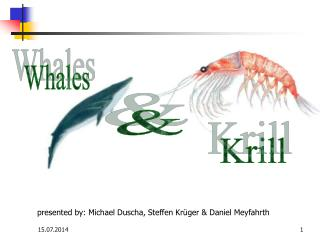DownloadDownload PresentationWhales

# Whales

Télécharger la présentation## Whales

- - - - - - - - - - - - - - - - - - - - - - - - - - - E N D - - - - - - - - - - - - - - - - - - - - - - - - - - -
##### Presentation Transcript

1. Whales & Krill presented by: Michael Duscha, Steffen Krüger & Daniel Meyfahrth

2. Whales and Krill Introduction • tow populations • differential equation • mathematical model

3. Whales and Krill Krill

4. Whales and Krill Whales Fisherman cometh

5. Whales and Krill Removing the dimensions • Reason : • Different units of measurements causes different numerical answers •  We don‘t know exact numbers of krill and whales [Krill / Krill] [Whales / ((Whales / Krill)*Krill)]

6. Whales and Krill Examination about C, alpha, K0 and r1 Dimensionsless

7. Whales and Krill Equilibriumpoints / Stability Trivial solution  x1= x2 = 0 Initial condition x1 > 0 ; x2 > 0 ; F1 and F2 < 1

8. Whales and Krill The effect of fishing F1 < 1 and F2 > 1 • Whale population decreases • Krill population increases more quickly or decreases more slowly F1 > 1 and F2 > 1 • Whale population decreases • Krill population will also decrease

9. Whales and Krill The effect of fishing Y1=r1*K*F1 and Y2=r2*W*F2 Y1*= max when F2 = 1  Y2* = 0 For fixed F2, the krill yield is maximized by letting F1 = 0.5 For fixed F1,  dY2* / dF2 = 0 The whale yield is maximized by:

10. Whales and Krill Total value [the best fishing policy] Is the relativ value of the whales and krill  Very large  Very small

11. Whales and Krill Conclusions Wanted: specific numerical solutions for F1 and F2 Problem: for this it would be necessary to measure:

12. Whales and Krill How can we measure the worth of an animale that is in danger of total extinction ??? How much do we care about preserving the biological heritage of this planet ???